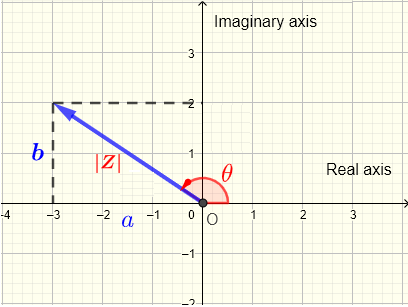# Modulus and Argument of a Complex Number - Calculator

 

An online calculator to calculate the modulus and argument of a complex number in standard form.

Let $Z$ be a complex number given in standard form by
$Z = a + i$
The modulus $|Z|$ of the complex number $Z$ is given by
$|Z| = \sqrt {a^2 + b^2}$
and the argument of the complex number $Z$ is angle $\theta$ in standard position.
Note
Since the above trigonometric equation has an infinite number of solutions (since $\tan$ function is periodic), there are two major conventions adopted for the rannge of $\theta$ and let us call them conventions 1 and 2 for simplicity.
Convention (1) define the argumnet $\theta$ in the range: $0 \le \theta \lt 2\pi$
Convention (2) defines the argument $\theta$ in the range : $(-\pi, +\pi ]$
The four quadrants , as defined in trigonometry, are determined by the signs of $a$ and $b$
If the terminal side of $Z$ is in quadrant (I) or (II) the two conventions give the same value of $\theta$.
If the terminal side of $Z$ is in quadrant (III) or (IV) convention one gives a positive angle and covention (2) gives a negative angle related by
$\theta_{\text{convention 2}} = \theta_{\text{convention 1}} - 2\pi$
This calculator calculates $\theta$ for both conventions.## Use of the calculator to Calculate the Modulus and Argument of a Complex Number

1 - Enter the real and imaginary parts of complex number $Z$ and press "Calculate Modulus and Argument". The outputs are the modulus $|Z|$ and the argument, in both conventions, $\theta$ in degrees and radians.

 $Z$   =   10     -10 $i$ Decimal Places = 3 Modulus: $|Z|$ = Argument in Radians $\theta$ = (convention 1) $\theta$ = (convention 2) Argument in Degrees $\theta$ = $^{\circ}$ (convention 1) $\theta$ = $^{\circ}$ (convention 2)

## Use the calculator of Modulus and Argument to Answer the Questions

1. Use the calculator to find the arguments of the complex numbers $Z_1 = -4 + 5 i$ and $Z_2 = -8 + 10 i$ . Why are they equal?
2. Find the arguments of the complex numbers $Z_1 = 3 - 9 i$ and $Z_2 = - 3 + 9i$. Why is the difference between the two arguments equal to $180^{\circ}$?
3. Find the ratio of the modulii of the complex numbers $Z_1 = 8 + 16 i$ and $Z_2 = 2 + 4 i$. Why is the ratio equal to $4$?
4. Find the ratio of the modulii of the complex numbers $Z_1 = - 8 - 16 i$ and $Z_2 = 2 + 4 i$. Why is the ratio equal to $4$?
5. Use the above results and other ideas to compare the modulus and argument of the complex numbers $Z$ and $k Z$ where $k$ is a real number not equal to zero.